#### You may also like### Fitting In

The largest square which fits into a circle is ABCD and EFGH is a square with G and H on the line CD and E and F on the circumference of the circle. Show that AB = 5EF. Similarly the largest equilateral triangle which fits into a circle is LMN and PQR is an equilateral triangle with P and Q on the line LM and R on the circumference of the circle. Show that LM = 3PQ### Look Before You Leap

Can you spot a cunning way to work out the missing length?### Triangle Midpoints

You are only given the three midpoints of the sides of a triangle. How can you construct the original triangle?

##### Age 14 to 16Challenge Level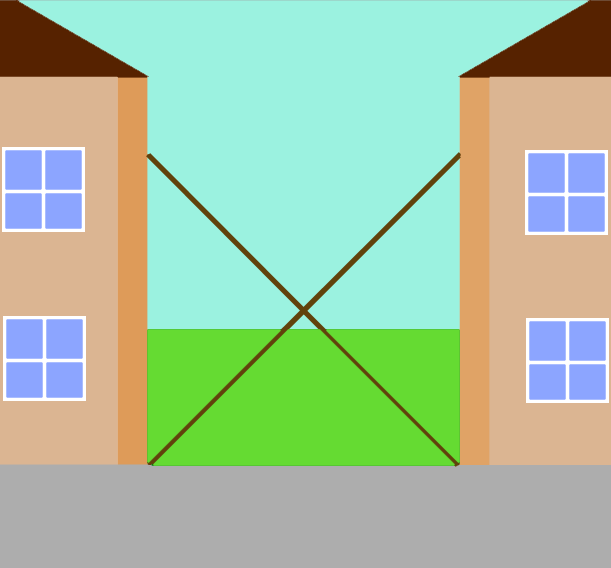The picture shows two ladders propped against facing walls. The end of the first ladder is $5\text{m}$ above the base of the second wall. The second ladder is $5\text{m}$ above the base of the first wall.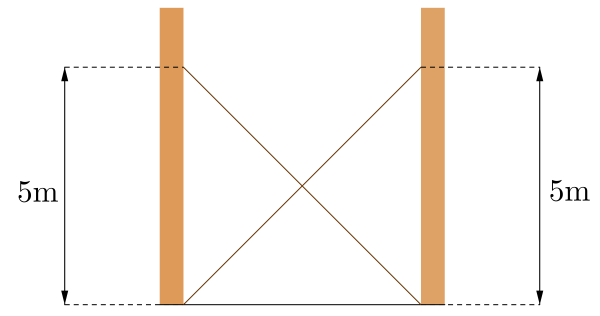Can you work out at what height the ladders cross?

Are you surprised that we don't need to know the distance between the two walls?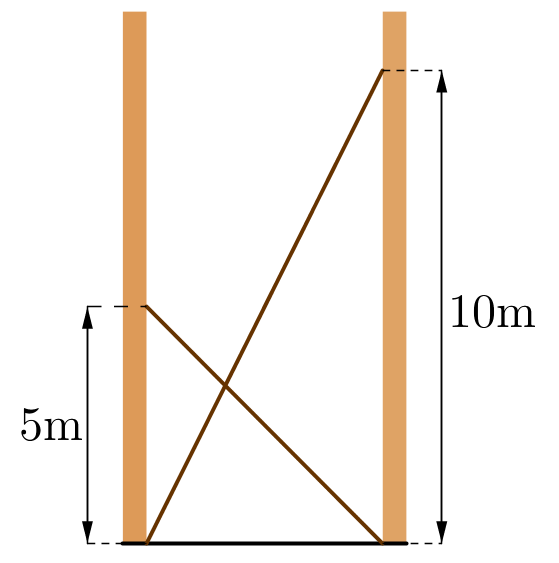Suppose now the first ladder is $10 \text{m}$ above the base of the second wall, with the second ladder remaining as before.

Can you work out at what height the ladders cross now?

Click below to reveal a diagram that may help you get started: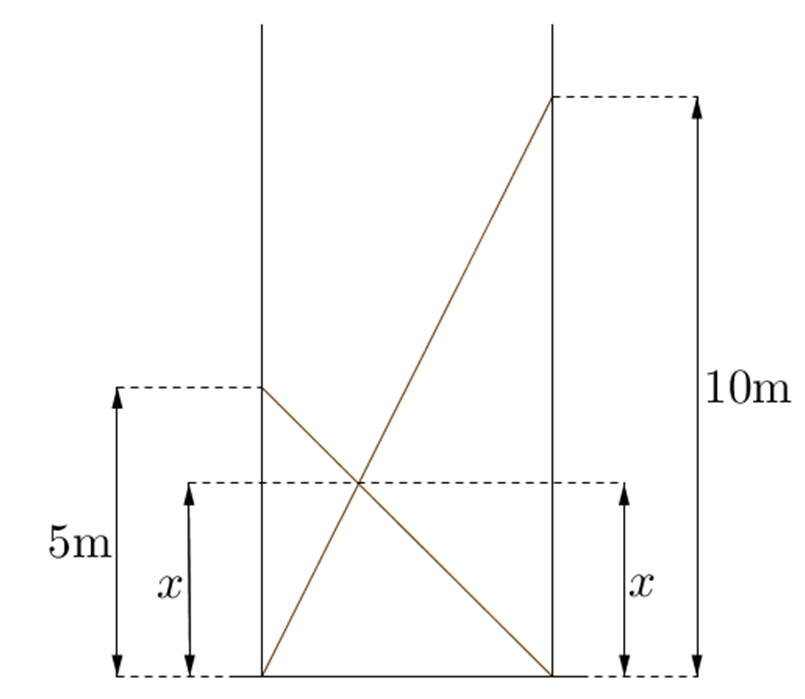Can you find any pairs of similar triangles in this diagram?
How do these help you to find the value of $x$?

Suppose the first ladder meets the wall at a height of $a$ metres, as in the diagram below.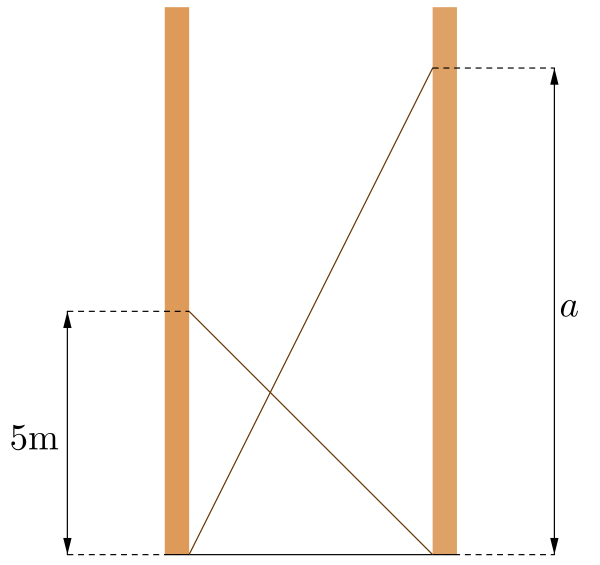At what height do the ladders cross now?

Suppose the second ladder meets the wall at a height of $b$ metres, as in the diagram below.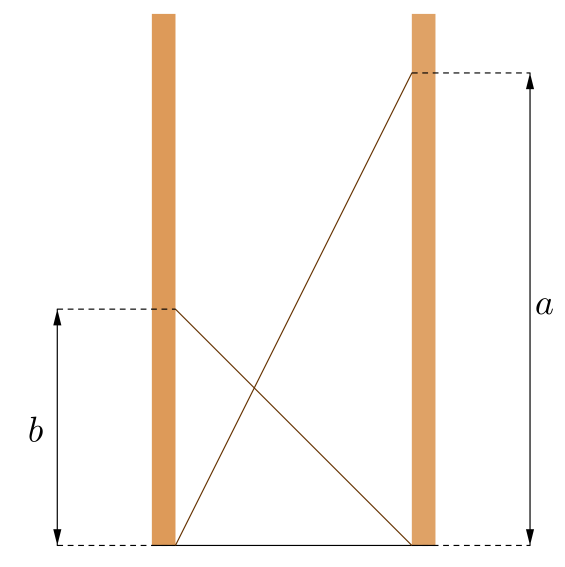At what height do the ladders cross now?